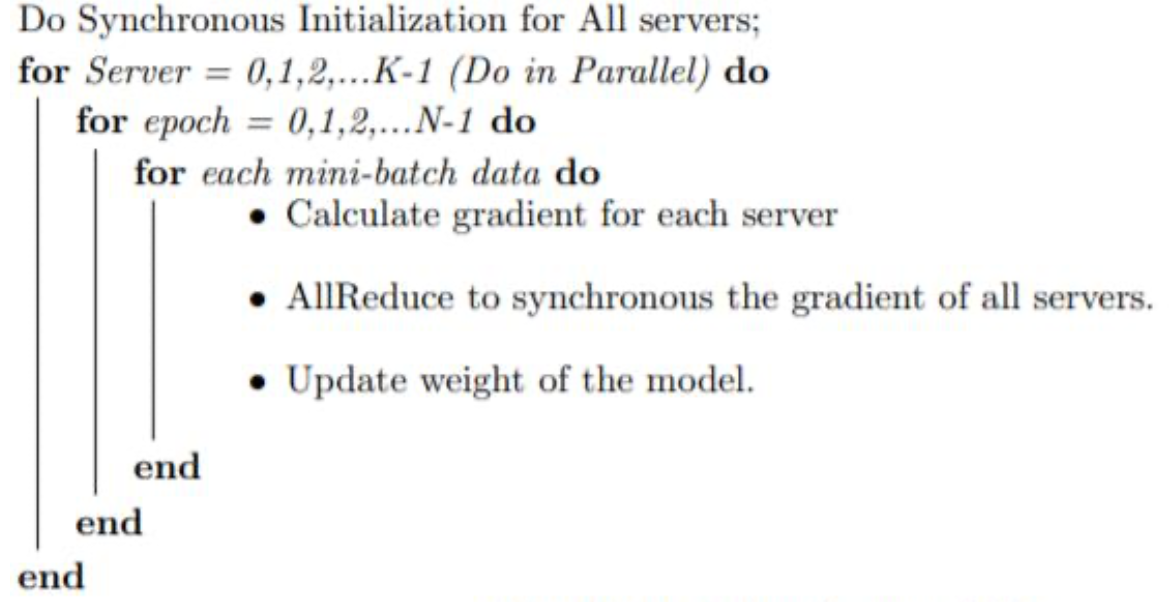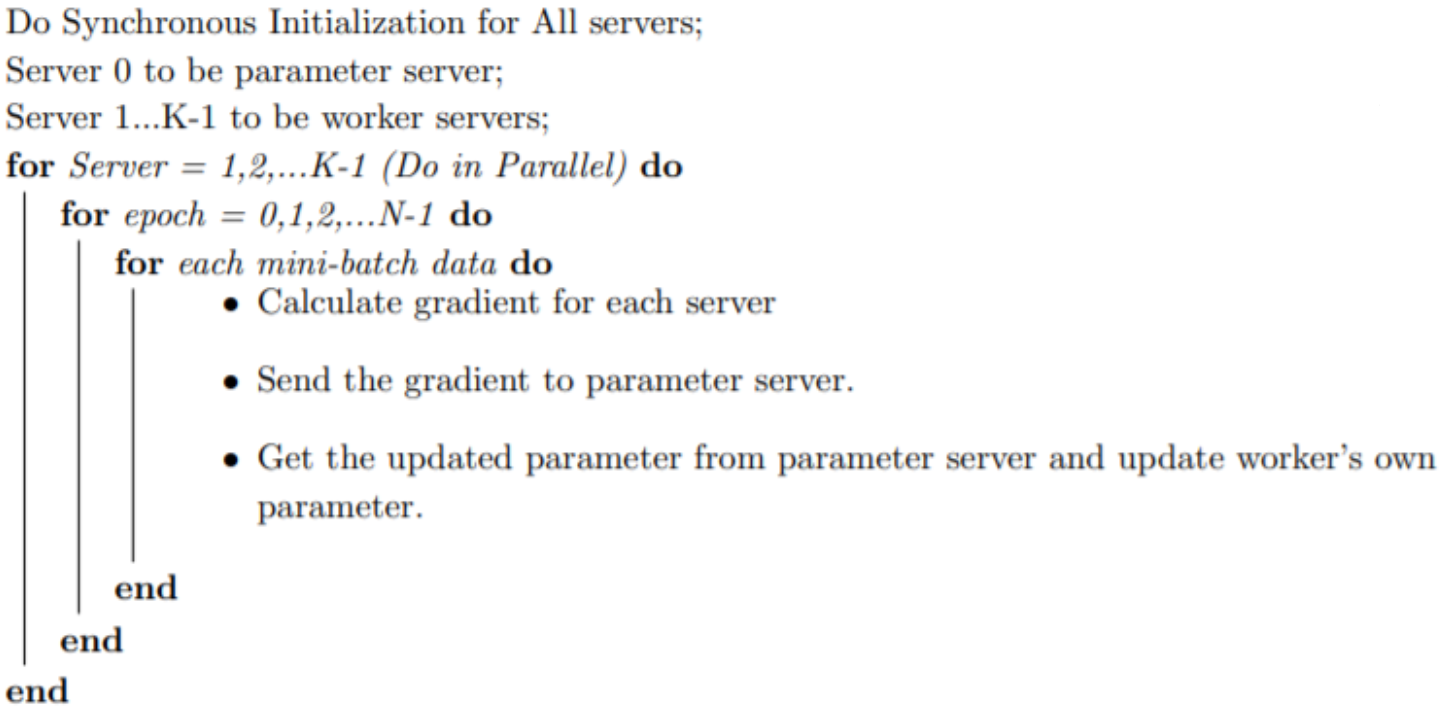# Distributed Deep Learning Patterns: Synchronous and Asynchorous SGD

In this post I’ll detail implementations of synchronous and asynchorous stochastic gradient descent (SSGD/ASGD) approaches for training deep learning models and the trade-offs. In the torch ecosystem, the DistributedDataParallel class does a pretty good job of sweeping the parallelization under the rug. Lightening is a third party helper library that also abstracts away the details of training more elaborate deep learning models like GANs or BERT on GPUs and TPUs.

The figure below gives an overview of the SSGD and ASGD algorithms. SSGD is the simplest of the two approaches, once gradients are calculated on each node, parameter gradients are moved from GPU memory back onto a shared memory resource. The average gradient is calculated and weights are updated. Data shards or the same copies of the data can be moved onto each node. The obvious downside to this method the heavy IO costs moving data from GPU memory onto the shared memory. In the code implementation of SSGD, the all_reduce method gathers and adds the gradients. The average is taken and transferred back to the GPU.Unlike SSGD, ASGD is a non-blocking method of performing SGD. The parameters live on a parameter server and gradients are updated whenever a worker node has finished a calculation. Because the updates are asynchronous, the result is biased

## MPI Backend Process Initialization

Preamble code if MPI is being used as a backend. In both algos, the model parameters are initialized synchronously between all nodes.

``````from mpi4py import MPI

cmd = "/sbin/ifconfig"
out, err = subprocess.Popen(cmd, shell=True, stdout=subprocess.PIPE,
stderr=subprocess.PIPE).communicate()
ip = str(out).split("inet addr:").split() #get node IP

name = MPI.Get_processor_name()
comm = MPI.COMM_WORLD
rank = comm.Get_rank()
num_nodes = int(comm.Get_size())

ip = comm.gather(ip)

if rank != 0: #rank 0 is the parameter node
ip = None

ip = comm.bcast(ip, root=0)

os.environ['MASTER_PORT'] = '2222'

backend = 'mpi'
dist.init_process_group(backend, rank=rank, world_size=num_nodes)

#parameter initialization
for param in model.parameters():
tensor0 = param.data
dist.all_reduce(tensor0, op=dist.reduce_op.SUM)
param.data = tensor0/np.sqrt(np.float(num_nodes))

model.cuda()

``````

``````for idx, data in enumerate(train_loader):
inputs, labels = data
inputs = inputs.cuda()
labels = labels.cuda()

outputs = net(inputs)
loss = criterion(outputs, labels)

#Get tensors from each worker using all_reduce
#Propagate the loss
for param in net.parameters():
dist.all_reduce(tensor0,op=dist.reduce_op.SUM)
tensor0 /= float(num_nodes)

loss.backward()
optimizer.step()

for param in model.parameters():
tensor0 = param.data
dist.all_reduce(tensor0, op=dist.reduce_op.SUM)
param.data = tensor0/np.sqrt(np.float(num_nodes))

model.cuda()

``````

``````
#init parameters on the parameter server
tensor_buffer = {}
for name,param in model.named_parameters():
tensor_buffer[name] = torch.zeros(param.data.shape).cpu()

criterion = nn.CrossEntropyLoss()
optimizer = torch.optim.RMSprop(model.parameters(), lr = learning_rate)
scheduler = torch.optim.lr_scheduler.StepLR(optimizer,step_size=10,gamma=0.5)

for epoch in range(num_epochs):
model.train()
for batch_idx, (X_train_batch, Y_train_batch) in enumerate(trainloader):
X_train_batch,Y_train_batch = X_train_batch.cuda(), Y_train_batch.cuda()
output = model(X_train_batch)
loss = criterion(output, Y_train_batch)
loss.backward()

#if not the parameter server
req = None
if rank != 0:
for name,param in model.named_parameters():
req = dist.isend(tensor=tensor0, dst=0)
req.wait()
for name,param in model.named_parameters():
req = dist.irecv(tensor=tensor_buffer[name], src=0)
param.data = tensor_buffer[name].cuda()
req.wait()
else:
#calculate parameters on the parameter server
for ii in range(1,num_nodes):
for name,param in model.named_parameters():
req = dist.irecv(tensor=tensor_buffer[name],src=ii)